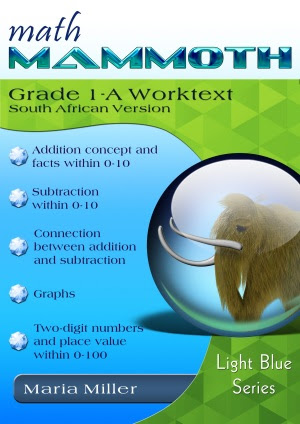## Posts

Showing posts from March, 2013

### Simplify a fraction multiplication before multiplyingI'm having difficulty in solving this question which involves calculating fractions - this question relates to finding an arc length. 140 divided by 360, multiplied by 2, multiplied by 22 divided by 7, multiplied by 12: 140 360 × 2 × 22 7 × 12 Solution: You can either put everything in the calculator, multiplying the top numbers, then dividing by 360 and 7. Or, you can simplify before you multiply. This process is actually quite handy! For example, the first fraction 140/360 can be simplified into 14/36, and then further into 7/18 before you multiply. We get 7 18 × 2 × 22 7 × 12 Now, the 7 in the numerator and the 7 in the denominator cancel out. Why? Every time we have the same number in the numerator and the denominator, and the only other operation involved is multiplication (like in our example), that number cancels out. It becomes the same situation as if you multiply by 7 and divide by 7: the result is 1. As a shortcut

### Math Mammoth South African versionThe South African version of Math Mammoth is now available for grades 1-3! You can either purchase any of the grade levels 1-3 separately, or purchase them all in a discounted bundle (33% off). The order page is here . Please see more information here: Math Mammoth Grade 1, South African version Math Mammoth Grade 2, South African version Math Mammoth Grade 3, South African version I hope South African homeschoolers and parents can spread the word!

### Common core concerns?

Questions about Math Mammoth and the Common Core Standards alignment? I made a new, hopefully comprehensive FAQ on this topic. Please refer to that document, and hopefully it will answer everyone's questions.

### How to tell your homeschool math program is workingDenise just posted an article How to Recognize a Successful Homeschool Math Program on her blog.  I enjoyed that a lot and recommend you read it too, no matter what math curriculum you are using! She summarizes it this way: If you are wondering how well your homeschool math program is working, pay attention to your children. Do they understand that common sense applies to math? Can they give logical reasons for their answers? Even when they get confused, do they know that math is nothing to fear? If so, then be assured: your children are already miles ahead of most of their peers. Their foundations are solid, and the details will eventually fall into place as you continue to play with mathematical ideas together. She also notes her 'yardstick' for measuring math anxiety : if your child does not fear word problems , he/she is not suffering from math anxiety. Photo by wecometolearn There was a time when my second daughter actually relished word

### Free worksheets for classifying triangles and quadrilateralsI've just completed making two new worksheet generators for HomeschoolMath.net: Classify triangles   - make worksheets for classifying triangles by their sides, angles, or both. Classify quadrilaterals   - make worksheets for classifying (recognizing, idenfitying, naming) quadrilaterals. There are seven special types of quadrilaterals: square, rectangle, rhombus, parallelogram, trapezoid, kite, scalene, and these worksheets ask students to name the quadrilaterals among these seven types. They look sort of like this: Use the links above to set your options (image size, number of problems, etc.) I had fun making the scripts, though also some challenges. But overall I enjoy such work - programming is similar to problem solving in math. In this case the problems often were math, such as how to make a script that gives me a kite or a scalene quadrilateraly with varying dimensions. I was using php GD library to first create a bunch of images, and then made the work

### Basic operations worksheets generator UPDATED!I have updated the Basic operations worksheet generator at HomeschoolMath.net—with new features! Now you can add a border around each problem, add additional workspace below the problem, and use a variable instead of an empty line. Even more: instead of choosing only one operation, you can also choose addition and subtraction, or multiplication and division. This worksheet generator makes printable worksheets both for whole numbers and integers , and for both horizontal and vertical form of operations. It is very versatile. You can make worksheets for... basic addition/subtraction facts mental addition and subtraction (for example, whole hundreds) multiplication tables, including missing factors basic division facts, including with remainders mental multiplication and division (for example, by powers of ten) column-form addition and subtraction, including subtraction with or without regrouping column-form multiplication (long multiplication) long division simp